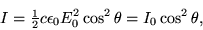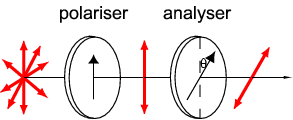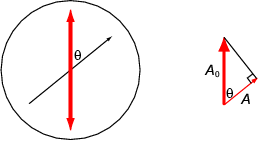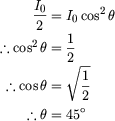# MALUS LAW

## Friday, September 02, 2005

MALUS LAW

The law giving the intensity produced when a polarizer is placed in front of an incident beam. In MKS,where c is the speed of light, is the permittivity of free space, is the magnitude of the electric field in the electromagnetic wave, and is the angle a polaroid analyzer is placed with respect to the polarizer.

POLARISER AND ANALYSEREarlier in this Section, the problem of a polariser acting on a polarised beam of light was introduced. We will now tackle this problem and calculate how much light is transmitted when the transmission axis of a Polaroid is at an angle to the plane of polarisation. The two cases illustrated in Figure 4 show what would happen if the transmission axis is parallel or perpendicular to the polarisation direction of the beam. In the former case all of the light is transmitted, in the latter case none of it is. Figure 6 shows what happens when the transmission axis of a sheet of Polaroid makes an angle with the plane of polarisation of an incident beam.

Figure 6 - Polarised beam incident on a second sheet of Polaroid
In Figure 6 an unpolarised beam of light is polarised by passing it through a sheet of Polaroid. The polarised beam is then passed through a second Polaroid sheet, often called the analyser. The transmission axis of the analyser makes an angle with the plane of polarisation of the incident beam. The beam that emerges from the analyser is polarised in the same direction as the transmission axis of the analyser.
The following animation shows how the intensity of the transmitted beam depends on the angle between the transmission axes of the polariser and the analyser.
A 'head-on' view of the analyser will help us to find the intensity of the transmitted beam (see Figure 7).

Figure 7 - Vertically polarised beam incident on an analyser
The incident beam has amplitude A 0. From Figure 7, the component of A 0 parallel to the transmission axis of the analyser is A 0cos. So the beam transmitted through the analyser has amplitude A, where

Equation 1
The intensity of a beam, measured in W m-2, is proportional to the square of the amplitude. Thus the intensity I 0 of the incident beam is proportional to A 0 2 and the intensity I of the transmitted beam is proportional to A 2 ( = (A 0cos)2). From Equation 1

Equation 2 is known as Malus' law, and gives the intensity of the beam transmitted through the analyser.
Example 1
A sheet of Polaroid is being used to reduce the intensity of a beam of polarised light. What angle should the transmission axis of the Polaroid make with the plane of polarisation of the beam in order to reduce the intensity of the beam by 50%?
We will use Malus' law to solve this problem, with I 0 as the intensity of the incident beam and I 0/2 as the intensity of the transmitted beam. Equation 2 then beco

POLARISATION AND MALUS LAW

polarization - law of extinction - Polaroid filters - vector components
What it shows: Polaroid filters absorb one component of polarization while transmitting the perpendicular components. The intensity of transmitted light depends on the relative orientation between the polarization direction of the incoming light and the polarization axis of the filter and is described quantitatively by Malus' cos2θ intensity law.
How it works: The demonstration apparatus is identical to that for the Polarization by Absorption demonstration. The Polaroid sheet in front of the light box (polarizer) and the (analyzer) Polaroid sheet are oriented so that their transmission axes are 90° with respect to each other. No light is transmitted by the analyzer -- no surprise. But now one adds a third Polaroid sheet between the polarizer and analyzer with its transmission axis oriented obliquely to the other two. The question is, does any light now emerge from the analyzer? Malus' law predicts that light will now emerge and the experiment confirms the prediction. Without the vector component analysis, it seems paradoxical that one can produce less absorption by the addition of more absorbers. Of course the paradox is resolved by the fact that these are selective absorbers, absorbing only the light whose polarization direction is perpendicular to its transmission axis.
Setting it up: Same as the Polarization by Absorption demonstration.
Comments: One could confirm Malus' law with just two Polaroid sheets (the polarizer and analyzer) and measure (with a light meter) the light intensity emerging from the analyzer as a function of angle. However, clear-cut quantitative results are spoiled by the fact that the analyzers are not perfect (significantly less than 50% transmission of unpolarized light) and this systematic error muddies the results. The three-polarizer experiment is a much more interesting way of demonstrating the effect, particularly if presented as a puzzle. For example, begin by inserting the third polarizer so that its axis is parallel with the first polarizer. Remove it and reinsert it parallel to the second (analyzer) polarizer. In both cases, nothing happens because the third polarizer is redundant to the first or second. Then surprise the audience by inserting it obliquely at 45°. Rating ***

main categories light/optics demos